# Calculus 3 : Derivatives

## Example Questions

1 2 3 4 5 6 7 8 9 11 Next →

### Example Question #101 : Derivatives

Find the derivative of the function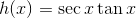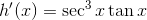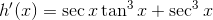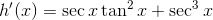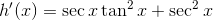Explanation:

To find the derivative of the function, we use the product rule: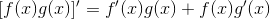with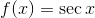and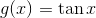so we get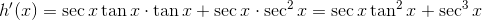### Example Question #102 : Derivatives

Find the derivative of the function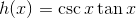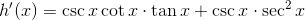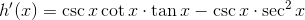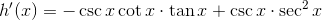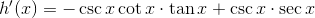Explanation:

To find the derivative of the function, we use the product rule:with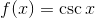andso we get### Example Question #103 : Derivatives

Find the derivative of the function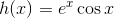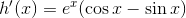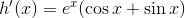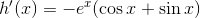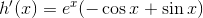Explanation:

To find the derivative of the function, we use the product rule:with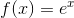and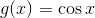so we get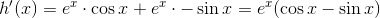### Example Question #104 : Derivatives

Find the derivative of the function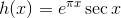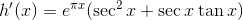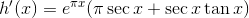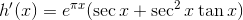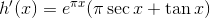Explanation:

To find the derivative of the function, we use the product rule:with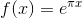and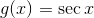so we get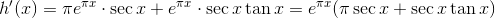### Example Question #105 : Derivatives

Find the derivative of the function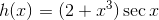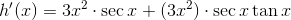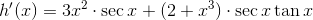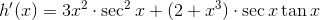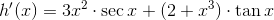Explanation:

To find the derivative of the function, we use the product rule:with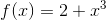andso we get### Example Question #106 : Derivatives

Find the derivative of the function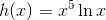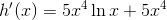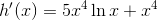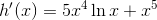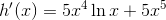Explanation:

To find the derivative of the function, we use the product rule:with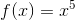and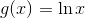so we get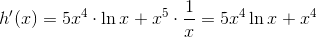### Example Question #107 : Derivatives

Find the derivative of the function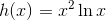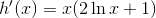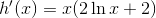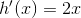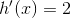Explanation:

To find the derivative of the function, we use the product rule:with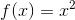andso we get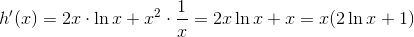1 2 3 4 5 6 7 8 9 11 Next →